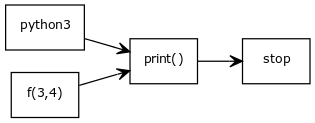To group sets of code you can use functions. Functions are small parts of repeatable code.
A function accepts parameters.

Without functions we only have a long list of instructions. Functions can help you organize code. Functions can also be reused, often they are included in modules.

Related course: Complete Python Programming Course & Exercises

## Example

### Functions

Functions can be seen as executable code blocks. A function can be used once or more.

A simple example of a function is:

The function is immediately called in this example. Function definitions always start with the def keyword.

Functions can be reusable, once created a function can be used in multiple programs. The print function is an example of that.

### Functions with parameters

In the example below we have parameter x and y. Type this program and save it as summation.py

In this example we have two functions: f(x,y) and print(). The function f(x,y) passed its output to the print function using the return keyword.### Return variables

Functions can return variables. Sometimes a function makes a calculation or has some output, this can be given to the program with a return varaible.

In many cases that output is stored in a variable:

In this case the program will call the function f with parameters 3 and 4, then save the output to the variable result.

If you are a beginner, then I highly recommend this book.

## Exercise

Try the exercises below

1. Make a function that sums the list mylist = [1,2,3,4,5]
2. Can functions be called inside a function?
3. Can a function call itself? (hint: recursion)
4. Can variables defined in a function be used in another function? (hint: scope)

After completing these continue with the next exercise.